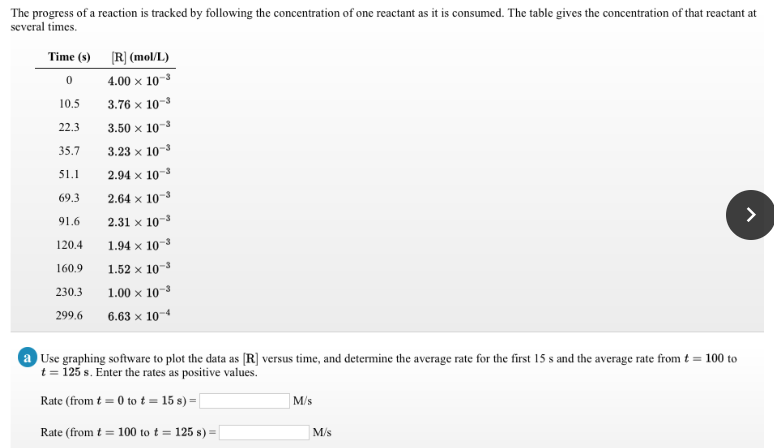# The progress of a reaction is tracked by following the concentration of one reactant as it is consumed. The table gives the concentration of that reactant at several times. Use graphing software to plot the data as [R] versus time, and determine the average rate for the first 15 s and the average rate from t = 100 to t = 125 s. Enter the rates as positive values. Rate (from t = 0 to t = 15 s) = ___ M/s Rate (from t = 100 to t = 125 s) = ___ M/s